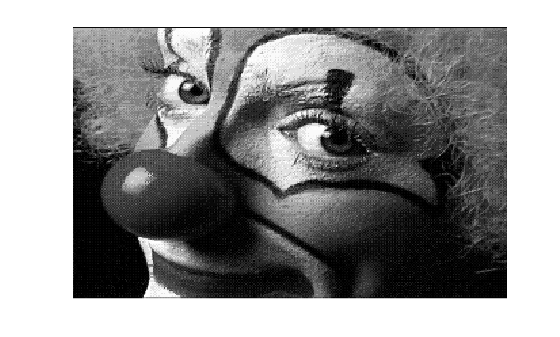# cmap2gray

Convert RGB colormap to grayscale colormap

## Syntax

``newmap = cmap2gray(map)``

## Description

example

````newmap = cmap2gray(map)` converts the RGB colormap `map` into the equivalent grayscale colormap `newmap`. ```

## Examples

collapse all

Load an indexed image with its colormap into the workspace. The `load` command returns a structure containing the image data and the colormap. View the image.

```indImage = load('clown.mat'); figure imshow(indImage.X,indImage.map)```Convert the RGB colormap that came with the image into a grayscale colormap.

`newmap = cmap2gray(indImage.map);`

Display the image with the grayscale colormap.

```figure; imshow(indImage.X,newmap)```## Input Arguments

collapse all

RGB colormap, specified as a c-by-3 numeric matrix with values in the range [0, 1]. Each row of `map` is a three-element RGB triplet that specifies the red, green, and blue components of a single color of the colormap.

If you have Parallel Computing Toolbox™ installed, `map` can also be a `gpuArray`.

Data Types: `double`

## Output Arguments

collapse all

Grayscale colormap, returned as a c-by-3 numeric matrix with values in the range [0, 1]. The three columns of `newmap` are identical, so that each row specifies a single intensity value.

If you have Parallel Computing Toolbox installed, then `newmap` can also be a gpuArray.

Data Types: `double`

## Extended Capabilities

### C/C++ Code GenerationGenerate C and C++ code using MATLAB® Coder™.

| | | (Image Processing Toolbox)

### Topics

Introduced in R2020b